# Molecule Polarity Chemistry Tutorial

## Key Concepts

• A non-polar molecule has no net dipole.

This can be achieved by the molecule having:

(a) only non-polar bonds

(b) polar bonds arranged symmetrically so that the dipoles cancel out

• A polar molecule has a net dipole.

This is achieved in the molecule by the molecule being made up of polar bonds arranged unsymmetrically so that the dipoles do not cancel out.

• To determine if a molecule is polar or non-polar, you will need to:

(a) use the electronegativity of atoms in each bond to decide if each bond is polar or non-polar

(b) determine the shape of the molecule using VSPER theory to decide if the bonds are arranged symmetrically (non-polar molecule) or not (non-polar molecule)

Please do not block ads on this website.
No ads = no money for us = no free stuff for you!

## Non-polar Molecules

A molecule is non-polar if there is no net dipole.

If a molecule is made up of atoms of the same element, then each covalent bond will be non-polar, so the overall molecule will be non-polar.
Example: H2

If a molecule is made up of atoms of different elements but with the same electronegativity, then the covalent bonds will be non-polar and the molecule will be non-polar.

Electronegativities of some commonly encountered elements are given below:

 Electronegativity Element trend 4.0 3.5 3.0 2.8 2.5 2.1 1.8 F O N Cl Br C S I P H Si most electronegative → least electronegative

Examples include1 NCl3

If a molecule is made up different elements which have different electronegativities, the molecule will be polar UNLESS the polar bonds are arranged symmetrically so that each dipole cancels out, in which case the molecule has a net dipole and is polar.
Symmetrical shapes of molecules include:

⚛ linear (bond angles 180°) for example CO2 and H2

⚛ trigonal planar (bond angles 120°) for example AlCl3

⚛ tetrahedral (bond angles 109.5°) for example CH4

⚛ octahedral (bond angles 90°) for example SF6

Worked examples of non-polar molecules:

Only non-polar bonds present
H2 H - H
(linear)
H2 is a non-polar molecule since both hydrogen atoms making up the molecule have equal electronegativity, 2.1 so there is no net dipole.

NCl3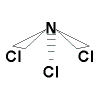(trigonal pyramidal)
NCl3 is a non-polar molecule since the nitrogen atom and the chlorine atoms making up the molecule have the same electronegativity (3.0) so there is no net dipole.1
Polar bonds arranged symmetrically
CO2 O = C = O
(linear)
Each C - O bond is polar since oxygen (3.5) is more electronegative than carbon (2.5), however, these bonds are arranged symmetrically (all angles are 180°) so that the two dipoles cancel out resulting in no net dipole for the molecule.

AlCl3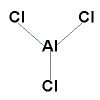(trigonal planar)
Each Al-Cl bond is polar since chlorine (3.0) is much more electronegative than aluminium (1.5), however, each Al-Cl bond in AlCl3 is arranged symmetrically (all angles are 120°) so that the dipoles cancel out resulting in no net dipole for the molecule.

CH4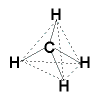(tetrahedral)
Each C-H bond is polar since carbon (2.5) is more electronegative than hydrogen (2.1), however, each C-H bond in CH4 is arranged symmetrically (all angles are 109.5°) so that the dipoles cancel out resulting in no net dipole for the molecule.

Do you know this?

Play the game now!

## Polar Molecules

A molecule will be polar if it has a net dipole.

A molecule will be polar if

(1) it is made up of elements with different electronegativities (example HCl)

AND also

(2) the covalent bonds are arranged unsymmetrically so that the dipoles do NOT cancle out

Examples of unsymmetrical shapes of molecules are:

⚛ bent (example H2O)

⚛ trigonal pyramidal (example NH3)

⚛ distortions of symmetrical shapes caused by substitution of atoms, for example distorted tetrahedron (CH3Cl) and distorted linear (HCN)

Worked examples of polar molecules:

Polar bonds arranged unsymmetrically
HCN
 δ+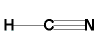δ- ------------> net dipole (linear)
Both the C-H and the C-N bonds are polar.
Nitrogen is more electronegative than carbon which is more electronegative than hydrogen.
The hydrogen atom takes on a partial positive (δ+) charge and the nitrogen takes on a partial negative charge (δ-).
This results in an unequal sharing of the bonding electrons resulting in a net dipole for molecule since the two dipoles do not cancel out.

H2O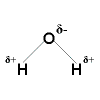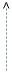netdipole (bent)
Each O-H bond is polar since oxygen is more electronegative than hydrogen so each hydrogen atom takes on a partial positive charge (δ+) and the oxygen atom takes on a partial negative charge (δ-).
The two O-H bonds are arranged unsymmetrically (angle between bonding pair < angle between bonding pair and lone pair < angle between lone pair and lone pair) resulting in a net dipole since the two dipoles do not cancel out.

NH3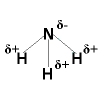netdipole (trigonal pyramidal)
Each N-H bond is polar since nitrogen is more electronegative than hydrogen so each hydrogen atom takes on a partial positive charge (δ+) and the nitrogen atom takes on a partial negative charge (δ-).
The three N-H bonds are arranged unsymmetrically (angle between bonding pairs < angle between bonding pairs and lone pair) resulting in a net dipole since the three dipoles do not cancel out.

CH3Cl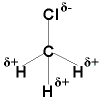netdipole (distorted tetrahedral)
Each C-H bond is polar since carbon is more electronegative that hydrogen, and the C-Cl bond is polar since chlorine is more elctronegative than either carbon or hydrogen.
Each hydrogen atom will take on a partial positve charge (δ+) and the chlorine atom will take on a partial negative charge (δ-) resulting in a net dipole since the dipoles will not cancel out owing to the difference in electronegativities of carbon, hydrogen and chlorine.

Do you understand this?

Take the test now!

1. Strictly speaking, the electronegativity of the nitrogen atom is slightly different to that of the chlorine atoms so the bonds are very slighly polar.
Since the bonds are arranged unsymmetrically as a result of the lone pair of electrons on the nitrogen atom, the molecule NCl3 is very slighly polar.